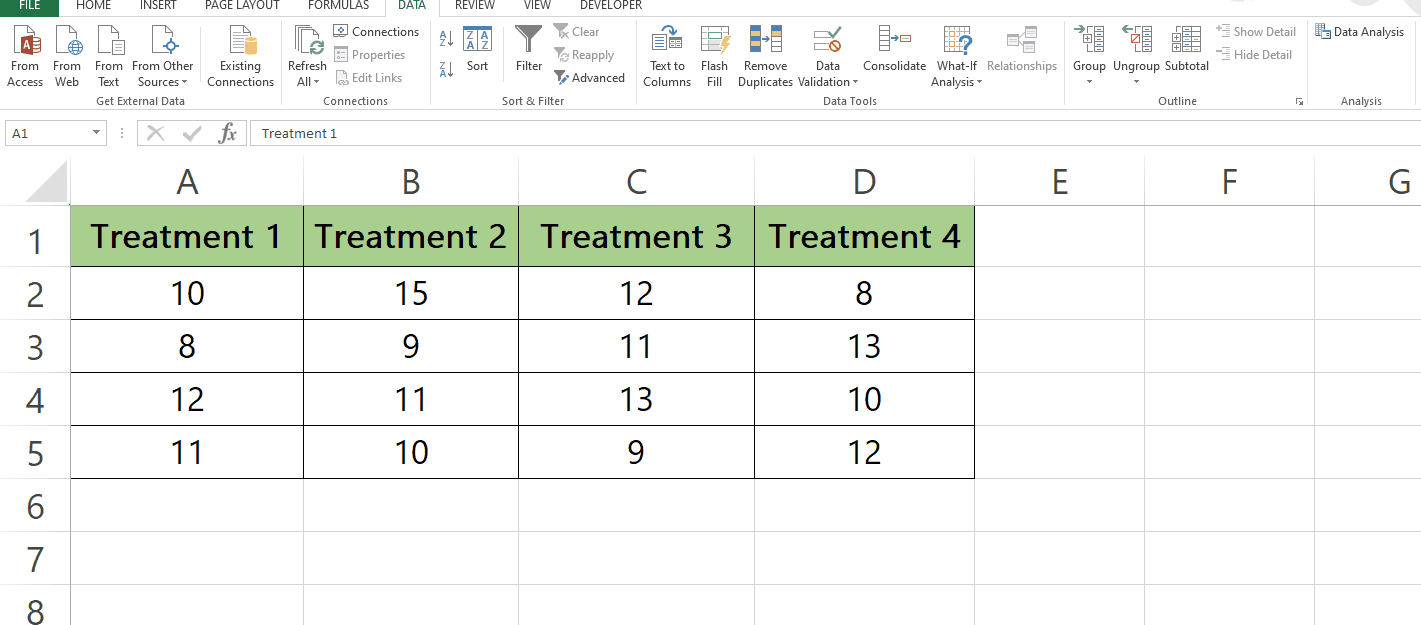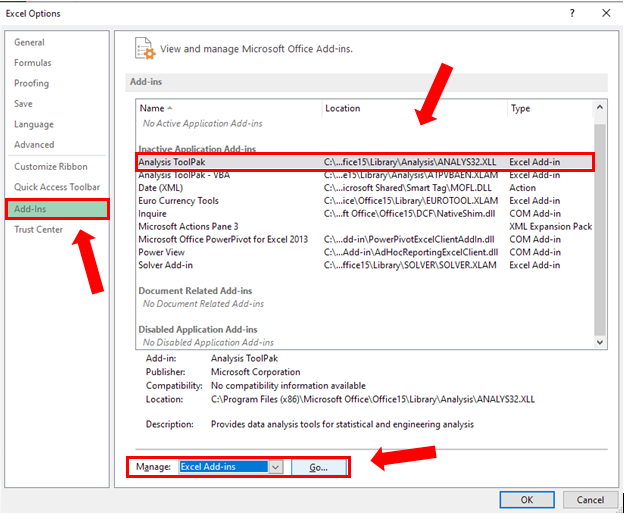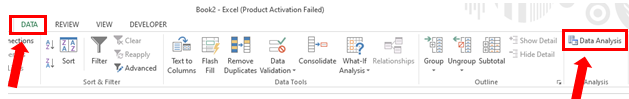# How to find F-statistic in excel.

Here we have a sample dataset that contains the results of an experiment where four different treatments were tested on a group of subjects. The values in columns represent the results for each treatment. In this tutorial, we learn how to perform F-statistic on the given data by following the simple steps below. Let’s have a look at the dataset first.The F-statistic is a widely used test statistic in statistical analysis, which helps to evaluate the equality of variances or means between two or more groups of data. It is a critical tool for testing hypotheses and making informed decisions based on statistical data. Microsoft Excel, with its built-in functions, provides an easy and efficient way to calculate the F-statistic, which is especially helpful for those who work with large datasets and want to analyze their data in a structured manner.

### Step – 1 Open Options.– First we have to enable the option of Data Analysis.
– Click on the File on the top left.
– Click on the options in the bottom left.

### Step – 2 Enabling the Data Analysis option.After you click on Options, the Excel Options menu will appear.
Choose Analysis ToolPak as shown below.
Then in the Manage box below make sure Excel Add-Ins is selected.
Click Go.

### Step – 3 Analysis ToolPak.Click OK.

### Step – 4 Open Data Analysis option.Now your Data analysis option has been enabled.
Click on the Data tab.
Click on Data Analysis in the Analysis group.

### Step – 5 Applying the F-test.On the Data Analysis menu there will be Analysis Tools.
Select F-Test Two-Sample for Variances option then click on OK.
In the F-Test Two-Sample for Variances menu, In the Input group select the Variable 1 and Variable 2 ranges.
In the Output Options select the output range as shown below.
Click OK.

## Conclusion:

The F-test is a statistical test used to compare the variances of two populations. In Excel, the F-test is commonly used to test the null hypothesis that two samples have the same variance.

The benefit of using the F-test in Excel is that it allows you to determine if two sets of data have a similar degree of variability. This information can be useful in a variety of contexts, such as quality control, experimental design, and data analysis.